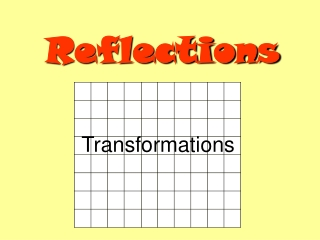DownloadDownload PresentationReflections

# Reflections

Download Presentation## Reflections

- - - - - - - - - - - - - - - - - - - - - - - - - - - E N D - - - - - - - - - - - - - - - - - - - - - - - - - - -
##### Presentation Transcript

1. Reflections Transformations

2. Starter Objective: Recap on vertical & horizontal lines.

3. Name the line y = 6 y = x X = 5 X = -1 Vertical lines are x = y = 1 Horizontal lines are y =

4. Lesson Objective: Reflect shapes in a given mirror line.

5. Reflect this triangle in the line x = 5

6. Check using tracing paper or a mirror

7. Reflect this rectangle in the line x = 1

8. Check using tracing paper or a mirror

9. Reflect this hexagon in the line x = 4

10. Check using tracing paper or a mirror

11. Reflect this rectangle in the line y = 2

12. Check using tracing paper or a mirror

13. Reflect this trapezium in the line y = 1

14. Check using tracing paper or a mirror

15. Reflect this trapezium in the line y = x

16. Check using tracing paper or a mirror

17. Name the line y = x + 4 y = x y = x + 1 y = -x + 3 y = -x

18. Reflect this triangle in the line y = - x

19. Check image using tracing paper

20. Reflect object in the line y = - x + 6

21. Check image using tracing paper

22. Challenge: Reflect the triangle in the y axis, then reflect that triangle in the line y = x.

23. Challenge: Reflect the triangle in the y axis. Then reflect that triangle in the line y = x. y = x

24. Challenge: Then reflect that triangle in the line y = x. y = x

25. Lesson Objective: Describing reflections. Draw and name the mirror line.

26. Draw in the mirror line and describe the reflection Reflection in the line x = 1

27. Draw in the mirror line and describe the reflection Reflection in the line y = 2

28. Draw in the mirror line and describe the reflection

29. Reflection in the line y = 3

30. Draw in the mirror line and describe the reflection

31. Reflection in the line x = 5

32. Draw in the mirror line and describe the reflection

33. Reflection in the x axis ( the line y = 0 )

34. Draw in the mirror line and describe the reflection

35. Reflection in the y axis ( the line x = 0 )

36. Draw in the mirror line and describe the reflection

37. Reflection in the line y = x

38. Draw in the mirror line and describe the reflection

39. Reflection in the line y = - x